### Affordable pricing from £10/month

In this worksheet, students will construct and measure bearings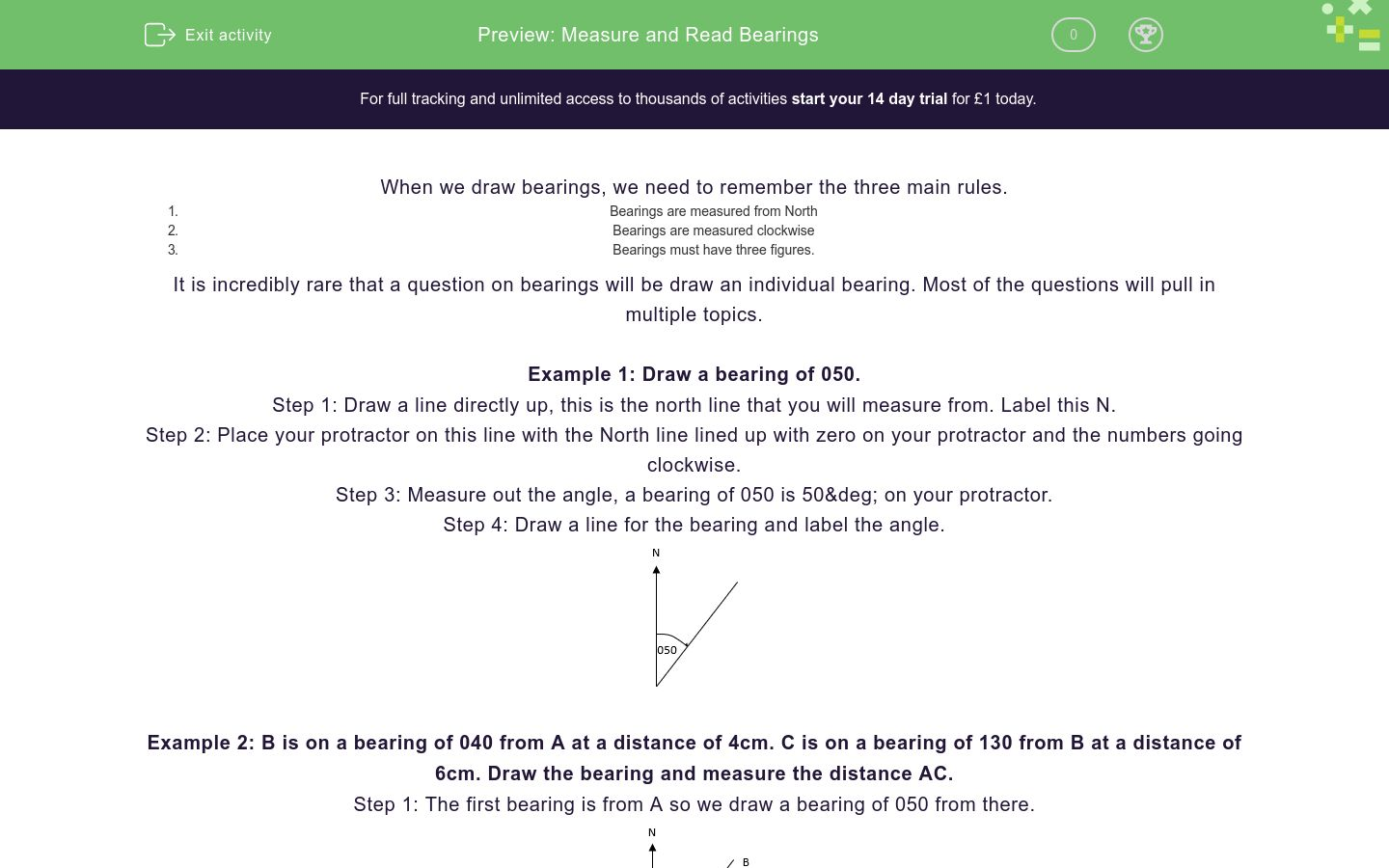Key stage:  KS 4

GCSE Subjects:   Maths

GCSE Boards:   AQA, Eduqas, Pearson Edexcel, OCR

Curriculum topic:   Geometry and Measures, Mensuration

Curriculum subtopic:   Mensuration and Calculation, Units and Measurement

Difficulty level:### QUESTION 1 of 10

When we draw bearings, we need to remember the three main rules.

1. Bearings are measured from North
2. Bearings are measured clockwise
3. Bearings must have three figures.

It is incredibly rare that a question on bearings will be draw an individual bearing. Most of the questions will pull in multiple topics.

Example 1: Draw a bearing of 050.

Step 1: Draw a line directly up, this is the north line that you will measure from. Label this N.

Step 2: Place your protractor on this line with the North line lined up with zero on your protractor and the numbers going clockwise.

Step 3: Measure out the angle, a bearing of 050 is 50&deg; on your protractor.

Step 4: Draw a line for the bearing and label the angle.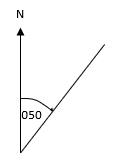Example 2: B is on a bearing of 040 from A at a distance of 4cm. C is on a bearing of 130 from B at a distance of 6cm. Draw the bearing and measure the distance AC.

Step 1: The first bearing is from A so we draw a bearing of 050 from there.Step 2: Draw another north line at B and measure the bearing of 130 from there.Step 3: Draw a line and measure from A to C.In this question, AC = 7.3cm. Don’t forget that you will get a margin of error if you are measuring. In this question, you would get the marks if you measured it as between 7.1 and 7.5 cm.

Example 3: B is 6cm directly east of A. Point C is 4cm from A and 5cm from point B. Draw a diagram and measure the bearing of C from A.

Step 1: We know that B is 6cm east from A, we can draw and label this.Step 2: Use a compass to show all the points that are 4cm from AStep 3: Use a compass to show all the points that are 5cm from B. The intersection of these two lines is point CStep 4: Draw a north line at A, a line from A to C and measure the bearingThe definition of bearings is that they are…

Which of these two diagrams shows a bearing of 050?Diagram A

Diagram B

Which of these two diagrams shows a bearing of 200?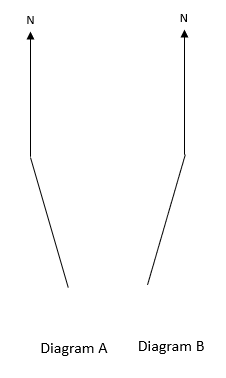Diagram A

Diagram B

A is 6cm from B on a bearing of 060. C is 7cm from B on a bearing of 135. Find the distance BC.

A is 2km from B on a bearing of 035. C is 4km from B on a bearing of 080. By drawing a diagram, find the distance BC.

B is 4cm on a bearing of 050 from A, C is 5cm on a bearing of 100 from B. Find the distance AC and the bearing of C from A.

Point B is 7cm directly east of A, Point C is 8cm from A on a bearing of 060.

Draw a diagram to find the distance BC.

Point B is 5cm directly east of A, Point C is 2cm from A on a bearing of 033.

Draw a diagram to find the distance BC.

B is 7cm directly east of A. C is 5cm from A and 4cm from B.

Find the bearing of C from A.

B is 5cm directly east of A. C is 4cm from A and 2cm from B.

Find the bearing of C from B.

• Question 1

The definition of bearings is that they are…

EDDIE SAYS
These are really important rules that underpin everything we do with bearings. Imagine if I said ‘turn 40 degrees’, would you know which way to go?
• Question 2

Which of these two diagrams shows a bearing of 050?Diagram A
EDDIE SAYS
Remember that bearings must be measured from NORTH. Diagram B is measured from east.
• Question 3

Which of these two diagrams shows a bearing of 200?Diagram B
EDDIE SAYS
200 is a greater than 180 so this must be a reflex angle. For a bearing, you need to go clockwise, this means that B must be correct as it is the reflex angle if you go clockwise. Diagram A is reflex if you go anticlockwise.
• Question 4

A is 6cm from B on a bearing of 060. C is 7cm from B on a bearing of 135. Find the distance BC.

10.7
10.8
10.9
11.0
11.1
EDDIE SAYS
If you have drawn this correctly, you should haveThe distance BC is 10.9 cm (+- 2mm)
• Question 5

A is 2km from B on a bearing of 035. C is 4km from B on a bearing of 080. By drawing a diagram, find the distance BC.

5.5
5.6
5.7
5.8
5.9
EDDIE SAYS
If you have drawn this correctly, you should haveThe distance BC is 10.9 cm (+- 2mm)
• Question 6

B is 4cm on a bearing of 050 from A, C is 5cm on a bearing of 100 from B. Find the distance AC and the bearing of C from A.

EDDIE SAYS
Drawing this situation will give the following diagram…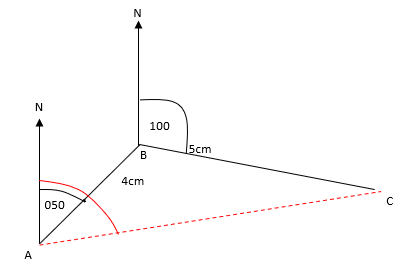Measuring AC will give 7.4cm (+-0.2) and measuring the bearing for C from A (In red) will give 080 (+-1)
• Question 7

Point B is 7cm directly east of A, Point C is 8cm from A on a bearing of 060.

Draw a diagram to find the distance BC.

3.9
4.1
4.0
4.2
4.3
EDDIE SAYS
If you draw this, you should get the diagram…One thing to be very careful here is that even though it looks like a right-angled triangle, we cannot assume that it is so all we can do is measure it.
• Question 8

Point B is 5cm directly east of A, Point C is 2cm from A on a bearing of 033.

Draw a diagram to find the distance BC.

4.1
4.2
4.3
4.4
4.5
EDDIE SAYS
If you draw this, you should get the diagram…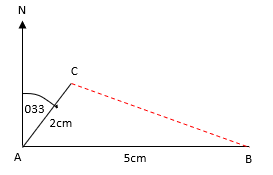Measuring BC will give a distance of 4.3(+-0.2) cm
• Question 9

B is 7cm directly east of A. C is 5cm from A and 4cm from B.

Find the bearing of C from A.

EDDIE SAYS
Remember that when you are measuring a bearing, you go from North (directly up on a diagram), go clockwise and give your answer as 3 figures.• Question 10

B is 5cm directly east of A. C is 4cm from A and 2cm from B.

Find the bearing of C from B.

EDDIE SAYS
Be careful here, the bearing here is a reflex one. The easiest way to do this is to measure the angle anticlockwise from North and then subtract it from 360°---- OR ----

Sign up for a £1 trial so you can track and measure your child's progress on this activity.

### What is EdPlace?

We're your National Curriculum aligned online education content provider helping each child succeed in English, maths and science from year 1 to GCSE. With an EdPlace account you’ll be able to track and measure progress, helping each child achieve their best. We build confidence and attainment by personalising each child’s learning at a level that suits them.

Get started